Random Number and Random Variate Generation 1 Random

• Slides: 45Random Number and Random Variate Generation 1Random Number and Random Variate Generation � How does the computer generate observations from various distributions specified after input analysis? � There are two main components to the generation of observations from probability distributions. 1. Random number generation. 2. Random variate generation. 2Random Number Generation � Random number generation – The generation of U(0, 1) random variates (observations from Uniform (0, 1) distribution). ◦ This serves as the foundation for the generation of observations from other distributions, which is called random variate generation. � Random Number Generator is the term used to describe the procedure and parameters used to generate the U(0, 1) observations. 3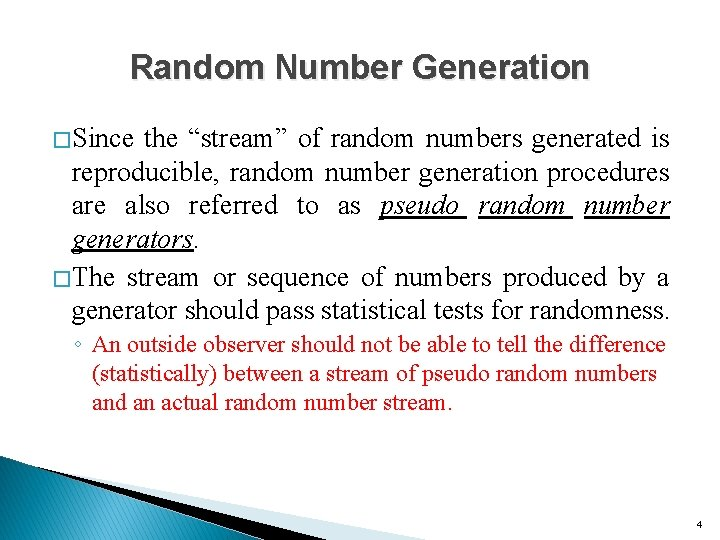Random Number Generation � Since the “stream” of random numbers generated is reproducible, random number generation procedures are also referred to as pseudo random number generators. � The stream or sequence of numbers produced by a generator should pass statistical tests for randomness. ◦ An outside observer should not be able to tell the difference (statistically) between a stream of pseudo random numbers and an actual random number stream. 4Pseudorandomness � A pseudorandom process appears random, but isn’t � Pseudorandom sequences exhibit statistical randomness ◦ but generated by a deterministic process � Pseudorandom sequences are easier to produce than a genuine random sequences � Pseudorandom sequences can reproduce exactly the same numbers ◦ useful for testing and fixing software. 5Pseudorandom Generator Seeds � Random number generators typically compute the next number in the sequence from the previous number � The first number in a sequence is called the seed ◦ to get a new sequence, supply a new seed (current machine time is useful) ◦ to repeat a sequence, repeat the seed 6Random Number Generation l Desirable Attributes: ◦ Uniformity ◦ Independence ◦ Efficiency ◦ Replicability ◦ Long Cycle Length 7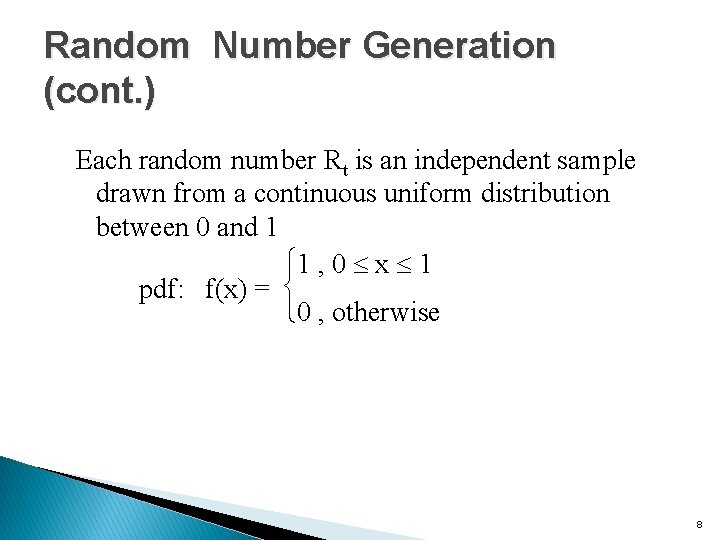Random Number Generation (cont. ) Each random number Rt is an independent sample drawn from a continuous uniform distribution between 0 and 1 ì 1 , 0 £ x £ 1 pdf: f(x) = í î 0 , otherwise 8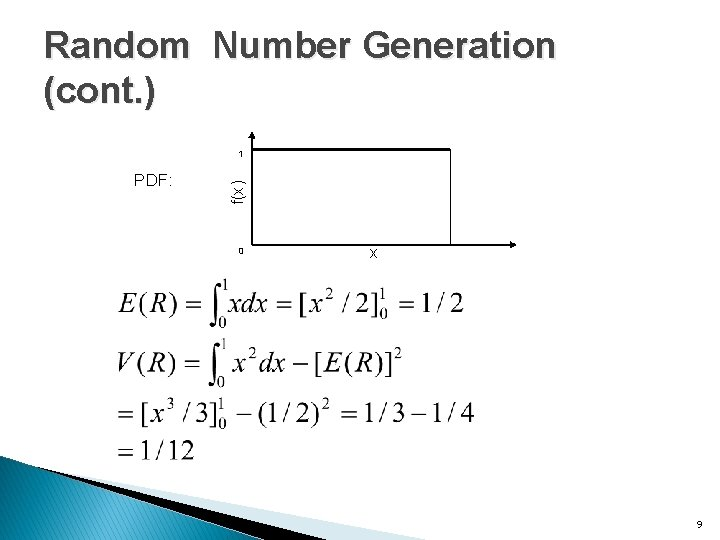Random Number Generation (cont. ) PDF: f(x) 1 0 x 9Random Number Generation � One early method – the midsquare method (von Neumann and Metropolis 1940) ◦ Start with a four digit positive integer Z 0. ◦ Square Z 0 to get an integer with up to eight digits (append zeros if less than eight). ◦ Take the middle four digits as the next four digit integer Z 1. ◦ Place a decimal point to the left of Z 1 to form the first “U(0, 1)” observation. ◦ Repeat 10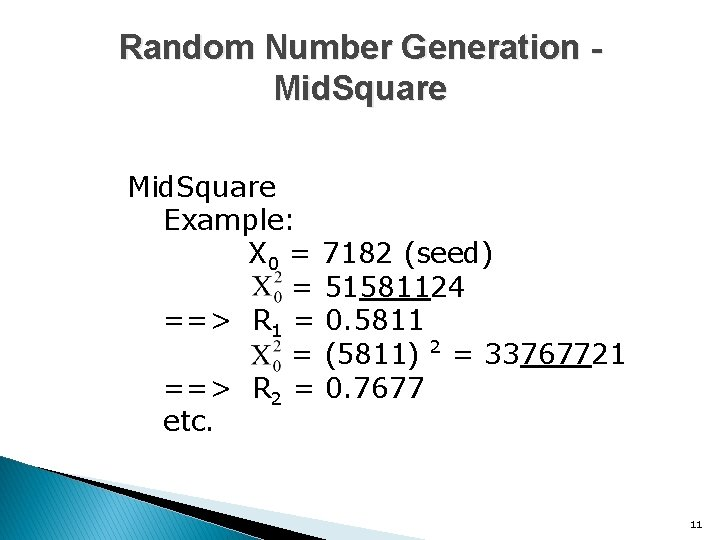Random Number Generation Mid. Square Example: X 0 = 7182 (seed) = 51581124 ==> R 1 = 0. 5811 = (5811) 2 = 33767721 ==> R 2 = 0. 7677 etc. 11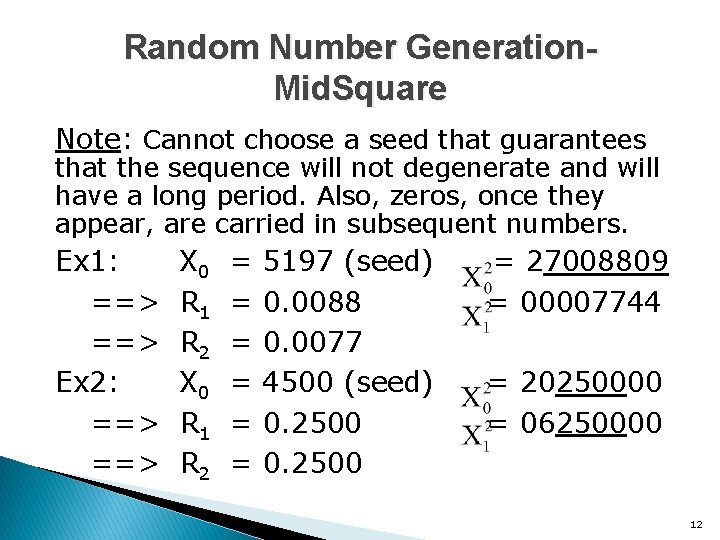Random Number Generation. Mid. Square Note: Cannot choose a seed that guarantees that the sequence will not degenerate and will have a long period. Also, zeros, once they appear, are carried in subsequent numbers. Ex 1: ==> Ex 2: ==> X 0 R 1 R 2 = = = 5197 (seed) 0. 0088 0. 0077 4500 (seed) 0. 2500 = 27008809 = 00007744 = 20250000 = 06250000 12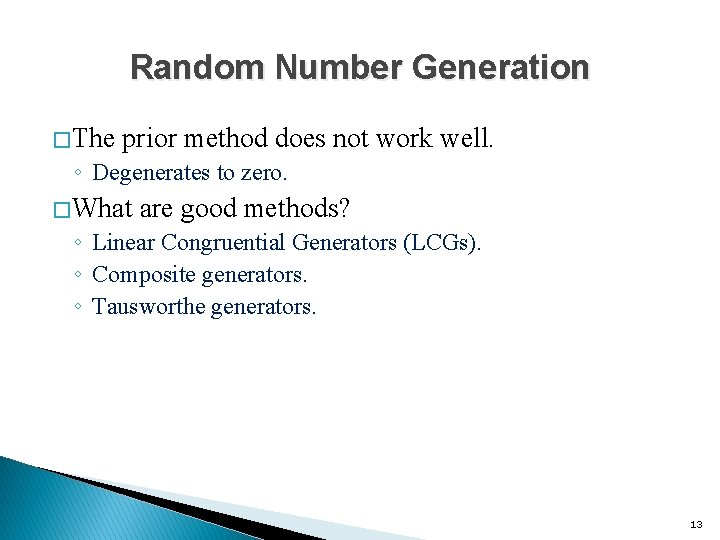Random Number Generation � The prior method does not work well. ◦ Degenerates to zero. � What are good methods? ◦ Linear Congruential Generators (LCGs). ◦ Composite generators. ◦ Tausworthe generators. 13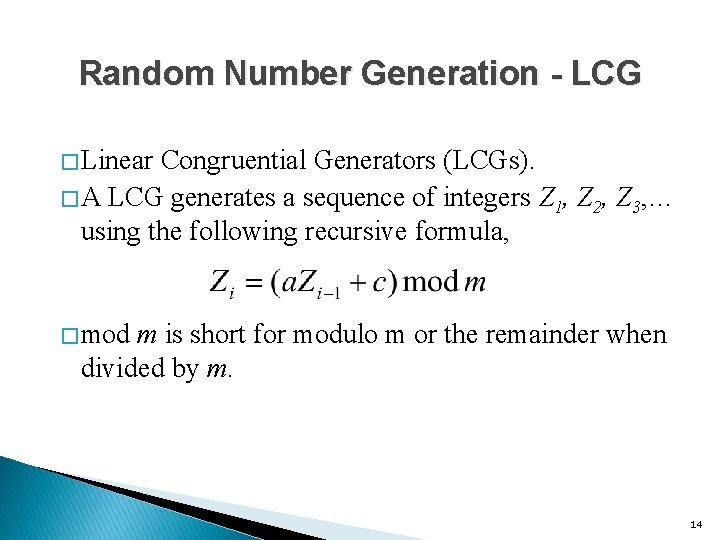Random Number Generation - LCG � Linear Congruential Generators (LCGs). � A LCG generates a sequence of integers Z 1, using the following recursive formula, Z 2, Z 3, … � mod m is short for modulo m or the remainder when divided by m. 14Random Number Generation - LCG � Since the mod m operation is used, all Zi’s will be between 0 and m-1. � To get the “U(0, 1)” random observations each Zi generated is divided by m. � So are the Ui’s really U(0, 1) random observations ? 15In-class Exercise � Let m=63, a=22, c=4 and Z 0 =19. ◦ Generate the first five “U(0, 1)” observations. 16Random Number Generation 17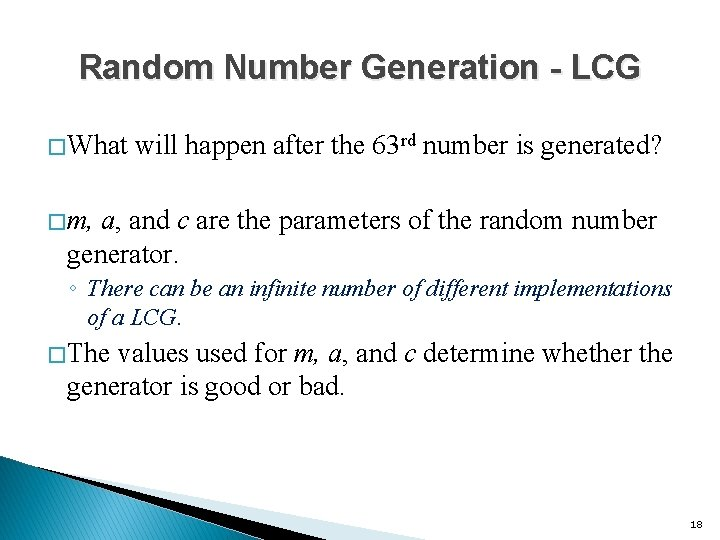Random Number Generation - LCG � What will happen after the 63 rd number is generated? � m, a, and c are the parameters of the random number generator. ◦ There can be an infinite number of different implementations of a LCG. � The values used for m, a, and c determine whether the generator is good or bad. 18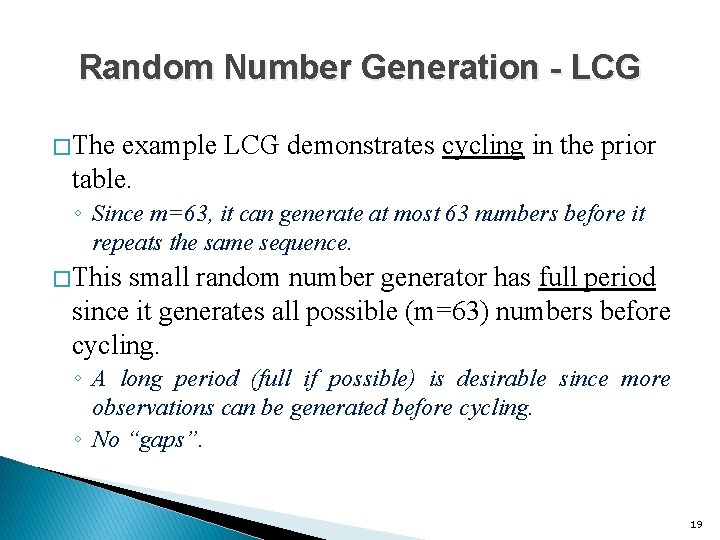Random Number Generation - LCG � The example LCG demonstrates cycling in the prior table. ◦ Since m=63, it can generate at most 63 numbers before it repeats the same sequence. � This small random number generator has full period since it generates all possible (m=63) numbers before cycling. ◦ A long period (full if possible) is desirable since more observations can be generated before cycling. ◦ No “gaps”. 19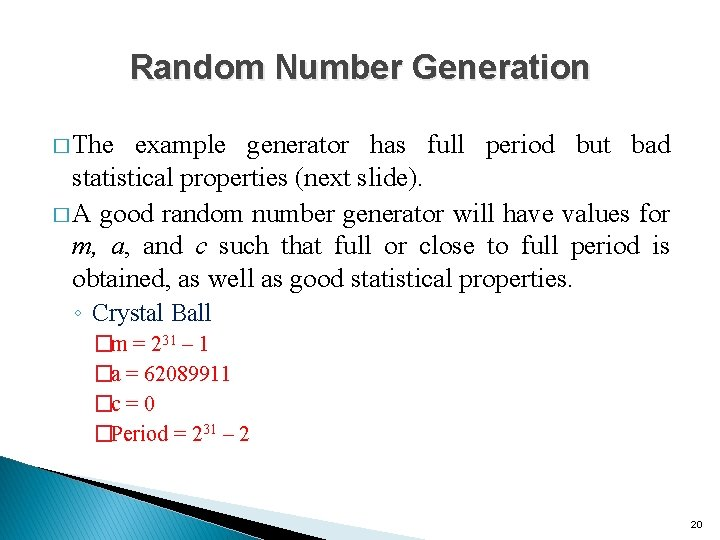Random Number Generation � The example generator has full period but bad statistical properties (next slide). � A good random number generator will have values for m, a, and c such that full or close to full period is obtained, as well as good statistical properties. ◦ Crystal Ball �m = 231 – 1 �a = 62089911 �c = 0 �Period = 231 – 2 20Random Number Generation - LCG � Theorem (Hull and Dobell 1962) ◦ The LCG Zi = (a. Zi-1 + c) mod m has full period if and only if the following three conditions hold. 1. The only positive integer that exactly divides both m and c is 1. 2. If q is a prime number that divides m, then q divides a-1. 3. If 4 divides m, then 4 divides a-1. � The parameters of the LCG dictate the period length of the LCG as well as other properties of the numbers generated. 21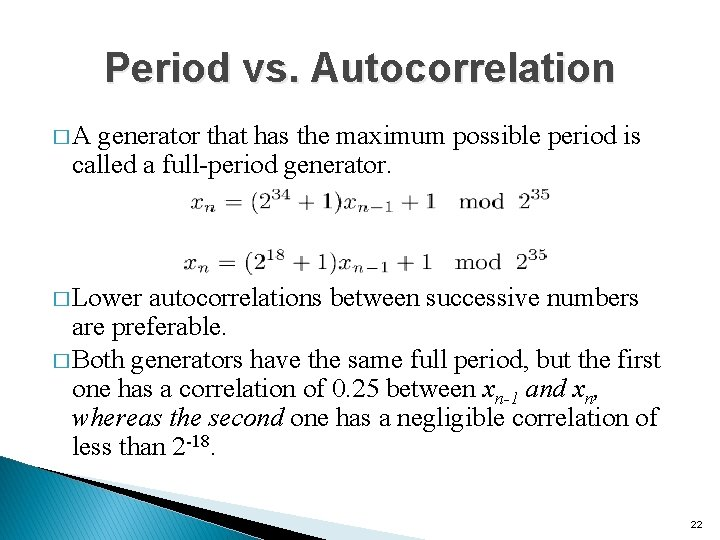Period vs. Autocorrelation � A generator that has the maximum possible period is called a full-period generator. � Lower autocorrelations between successive numbers are preferable. � Both generators have the same full period, but the first one has a correlation of 0. 25 between xn-1 and xn, whereas the second one has a negligible correlation of less than 2 -18. 22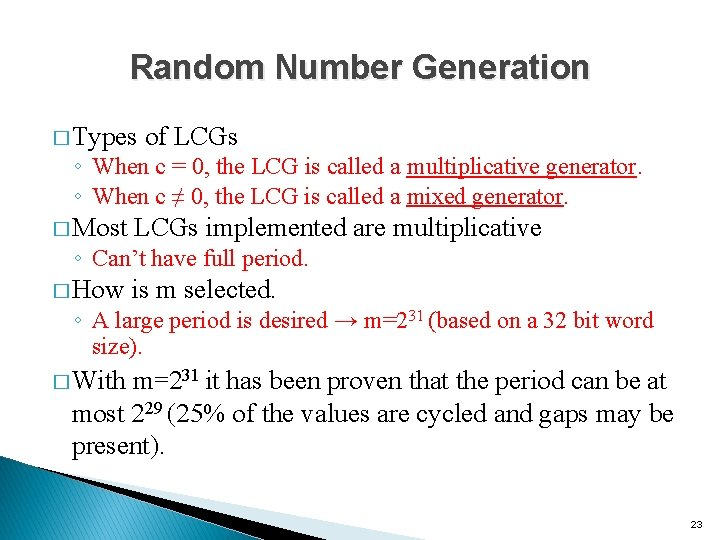Random Number Generation � Types of LCGs ◦ When c = 0, the LCG is called a multiplicative generator. ◦ When c ≠ 0, the LCG is called a mixed generator. � Most LCGs implemented are multiplicative ◦ Can’t have full period. � How is m selected. ◦ A large period is desired → m=231 (based on a 32 bit word size). � With m=231 it has been proven that the period can be at most 229 (25% of the values are cycled and gaps may be present). 23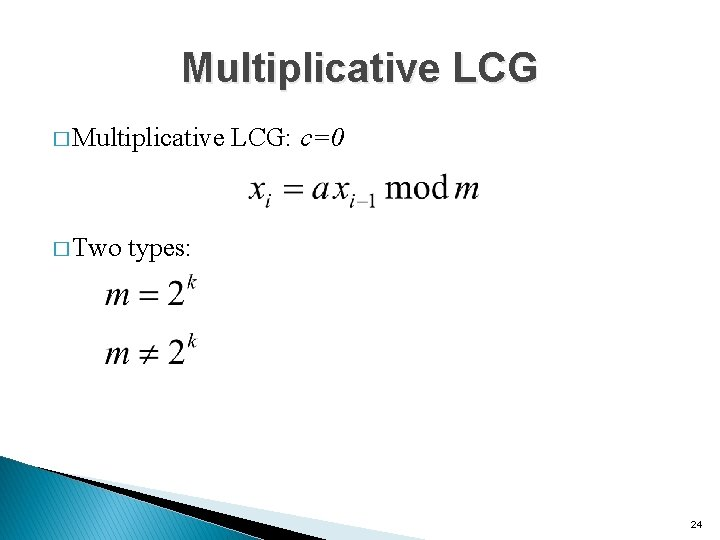Multiplicative LCG � Multiplicative LCG: c=0 � Two types: 24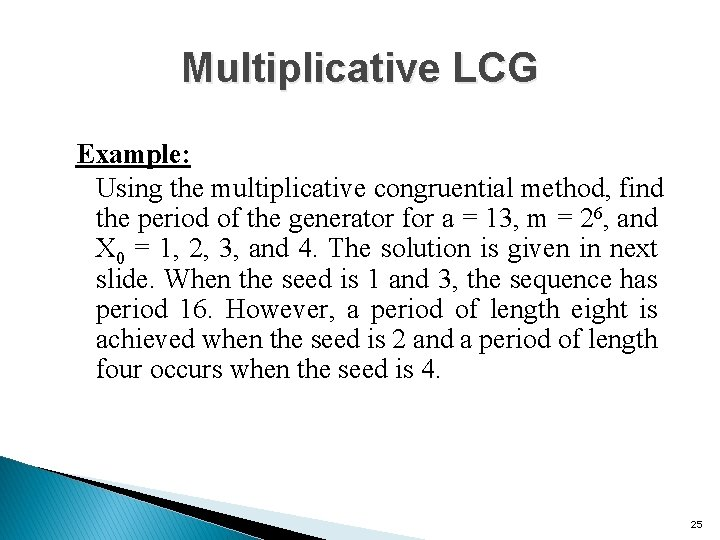Multiplicative LCG Example: Using the multiplicative congruential method, find the period of the generator for a = 13, m = 26, and X 0 = 1, 2, 3, and 4. The solution is given in next slide. When the seed is 1 and 3, the sequence has period 16. However, a period of length eight is achieved when the seed is 2 and a period of length four occurs when the seed is 4. 25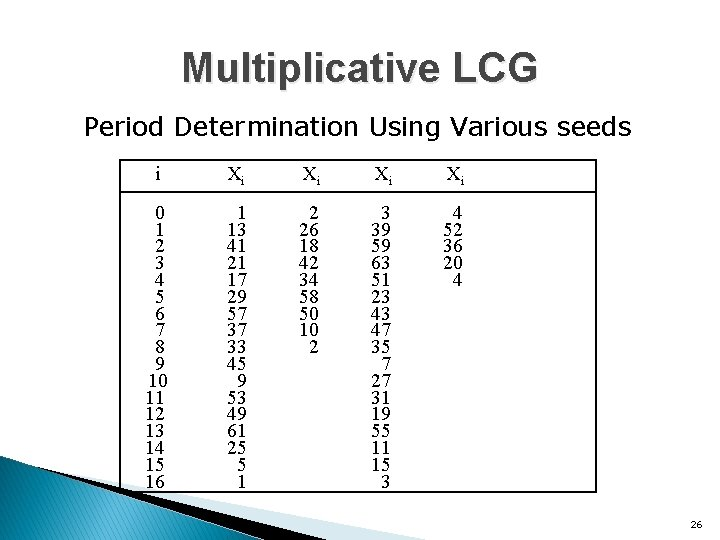Multiplicative LCG Period Determination Using Various seeds i 0 1 2 3 4 5 6 7 8 9 10 11 12 13 14 15 16 Xi Xi 1 13 41 21 17 29 57 37 33 45 9 53 49 61 25 5 1 2 26 18 42 34 58 50 10 2 3 39 59 63 51 23 43 47 35 7 27 31 19 55 11 15 3 4 52 36 20 4 26Multiplicative LCG with m=2 k � Maximum possible period 2 k-2 � Period achieved if multiplier a is of the form 8 i± 3, and the initial seed is an odd integer � One-fourth the maximum possible may not be too small � Low order bits of random numbers obtained using multiplicative LCG's with m=2 k have a cyclic pattern. 27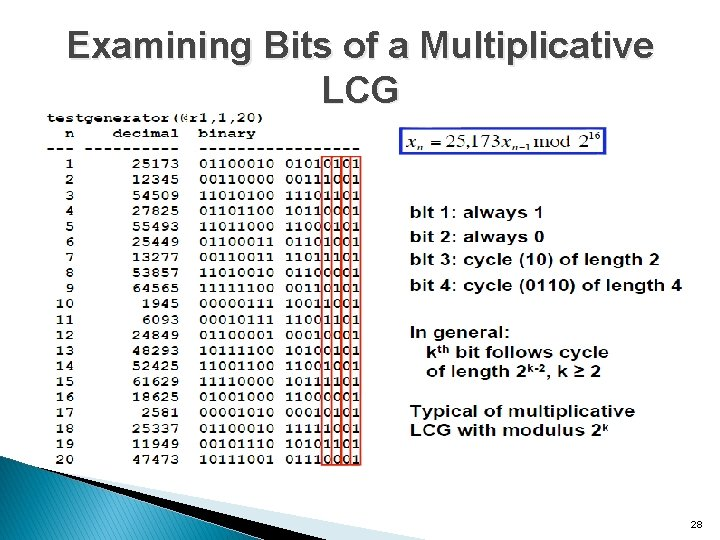Examining Bits of a Multiplicative LCG 28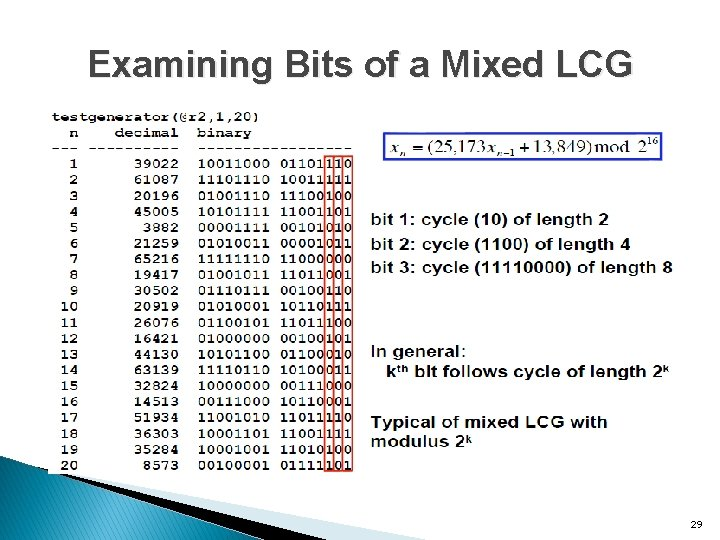Examining Bits of a Mixed LCG 29Multiplicative LCG with m≠ 2 k � When the modulus m is a prime number and a >1, the maximum period is m-1, no matter whether c=0 or not. � The maximum period m-1 is obtained if and only if ‘a’ is a primitive element modulo m. � If m is prime then ‘a’ is a primitive element modulo m (or primitive root of m) if and only if an mod m ≠ 1 for n=1, 2, 3, …, m-2. � Recommended. (Prime moduli are best in terms of sequence randomness. ) 30Multiplicative LCG with m≠ 2 k Example: � Starting with a seed of x 0=1: 1, 3, 9, 27, 19, 26, 17, 20, 29, 25, 13, 8, 24, 10, 30, 28, 22, 4, 12, 5, 14, 11, 2, 6, 18, 23, 7, 21, 1, … The period is 30 ⇒ 3 is a primitive root of 31 � With a multiplier of a = 5: 1, 5, 25, 1, … The period is only 3 ⇒ 5 is not a primitive root of 31 � Primitive roots of 31= 3, …? ? ? ? . 31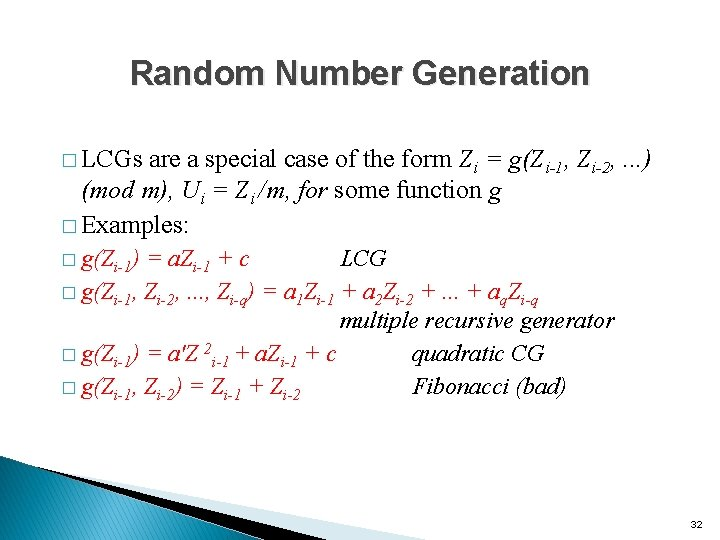Random Number Generation � LCGs are a special case of the form Zi = g(Zi-1, Zi-2, . . . ) (mod m), Ui = Zi /m, for some function g � Examples: � g(Zi-1) = a. Zi-1 + c LCG � g(Zi-1, Zi-2, . . . , Zi-q) = a 1 Zi-1 + a 2 Zi-2 +. . . + aq. Zi-q multiple recursive generator � g(Zi-1) = a'Z 2 i-1 + a. Zi-1 + c quadratic CG � g(Zi-1, Zi-2) = Zi-1 + Zi-2 Fibonacci (bad) 32Random Number Generation Composite Generators Combine two (or more) individual generators in some way. � Differencing LCGs ◦ Z 1 i and Z 2 i from LCGs with different moduli ◦ Let Zi = (Z 1 i – Z 2 i ) (mod m); Ui = Zi / m ◦ Very good statistical properties ◦ Very portable (micros, different languages) � Wichmann/Hill ◦ Use three LCGs to get U 1 i, U 2 i, and U 3 i sequences ◦ Let Ui = fractional part of U 1 i + U 2 i + U 3 i ◦ Long period, good statistics, portability 33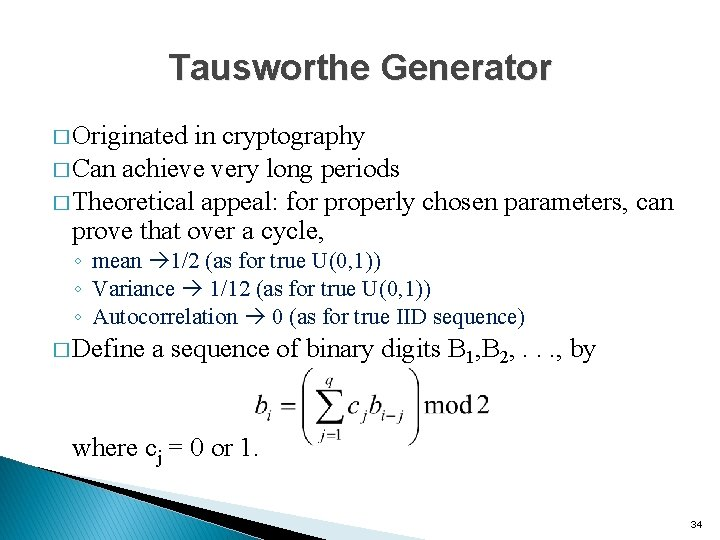Tausworthe Generator � Originated in cryptography � Can achieve very long periods � Theoretical appeal: for properly chosen parameters, can prove that over a cycle, ◦ mean 1/2 (as for true U(0, 1)) ◦ Variance 1/12 (as for true U(0, 1)) ◦ Autocorrelation 0 (as for true IID sequence) � Define a sequence of binary digits B 1, B 2, . . . , by where cj = 0 or 1. 34Tausworthe Generator � Looks a bit like a generalization of LCG’s. � Let D = delay operator such that Db(n)=b(n+1) � or � Since in mod 2 arithmetic subtraction is equivalent to addition, the preceding equation is equivalent to 35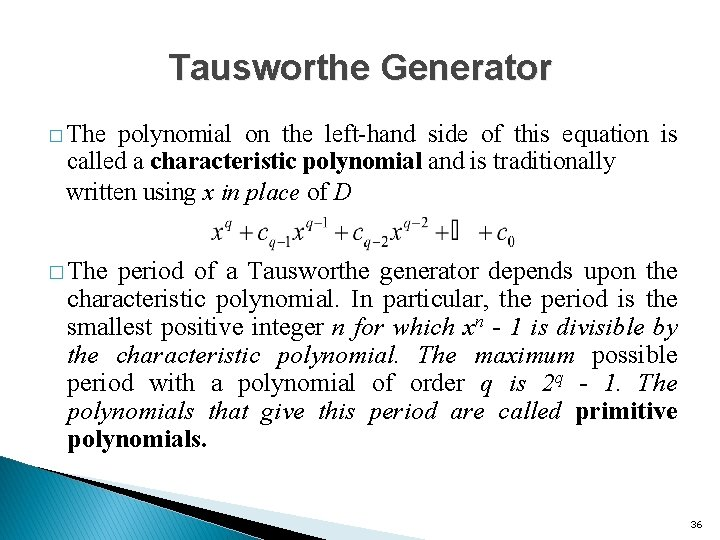Tausworthe Generator � The polynomial on the left-hand side of this equation is called a characteristic polynomial and is traditionally written using x in place of D � The period of a Tausworthe generator depends upon the characteristic polynomial. In particular, the period is the smallest positive integer n for which xn - 1 is divisible by the characteristic polynomial. The maximum possible period with a polynomial of order q is 2 q - 1. The polynomials that give this period are called primitive polynomials. 36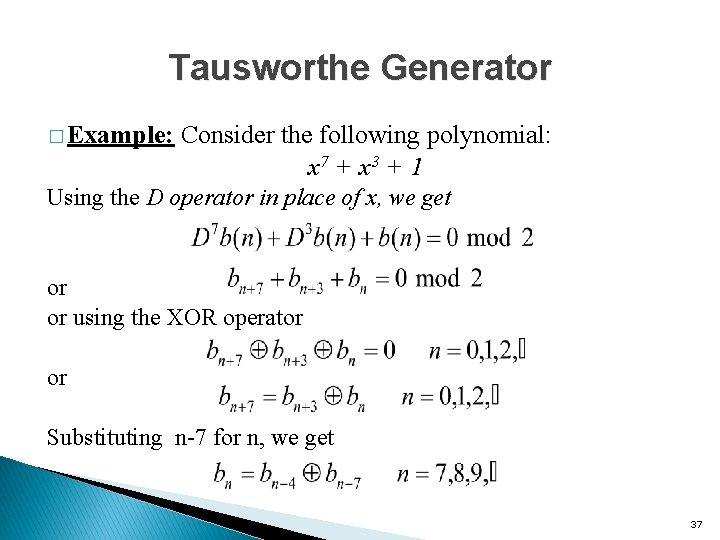Tausworthe Generator � Example: Consider the following polynomial: x 7 + x 3 + 1 Using the D operator in place of x, we get or or using the XOR operator or Substituting n-7 for n, we get 37Tausworthe Generator Starting with b 0 = b 1 =. . . = b 6 = 1, we get the following bit sequence: 38Tausworthe Generator � The complete sequence is: 1111111 0000111100 1011001 0010000 0010001 0011000 1011101 0110110 0000110101 0011100 1111011 0100001 0101011 111010001 1011100 0111111 1000000. � Period = 127 or 27 -1 bits ⇒ The polynomial x 7+x 3+1 is a primitive polynomial. 39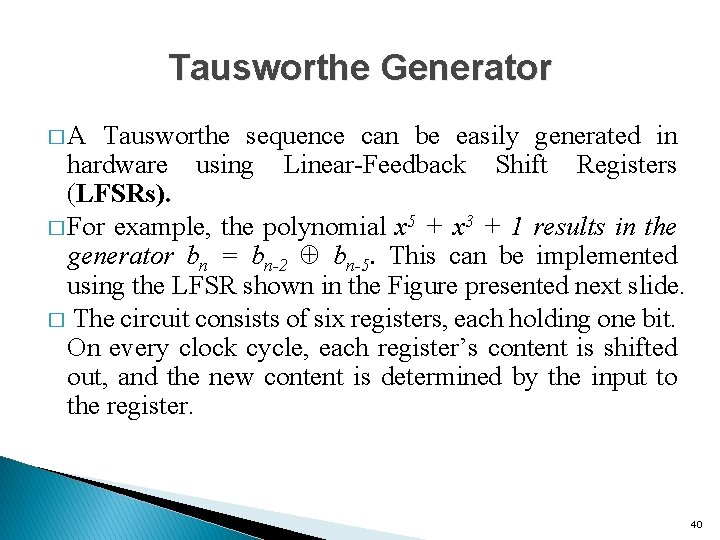Tausworthe Generator � A Tausworthe sequence can be easily generated in hardware using Linear-Feedback Shift Registers (LFSRs). � For example, the polynomial x 5 + x 3 + 1 results in the generator bn = bn-2 bn-5. This can be implemented using the LFSR shown in the Figure presented next slide. � The circuit consists of six registers, each holding one bit. On every clock cycle, each register’s content is shifted out, and the new content is determined by the input to the register. 40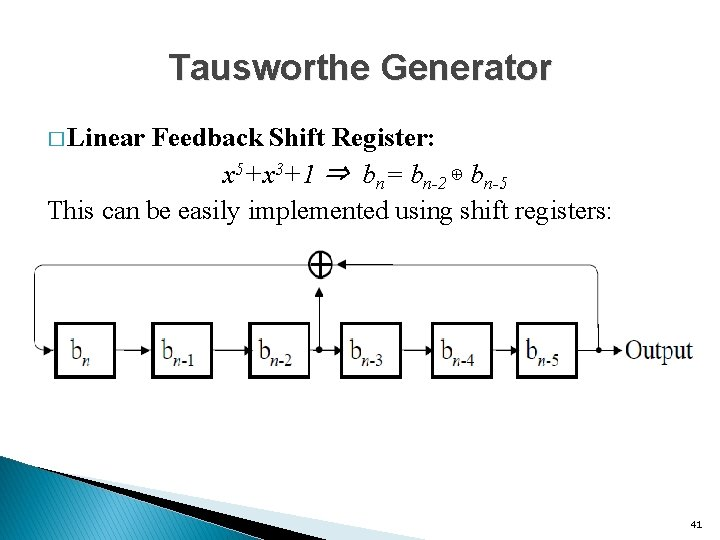Tausworthe Generator � Linear Feedback Shift Register: x 5+x 3+1 ⇒ bn= bn-2 ⊕ bn-5 This can be easily implemented using shift registers: 41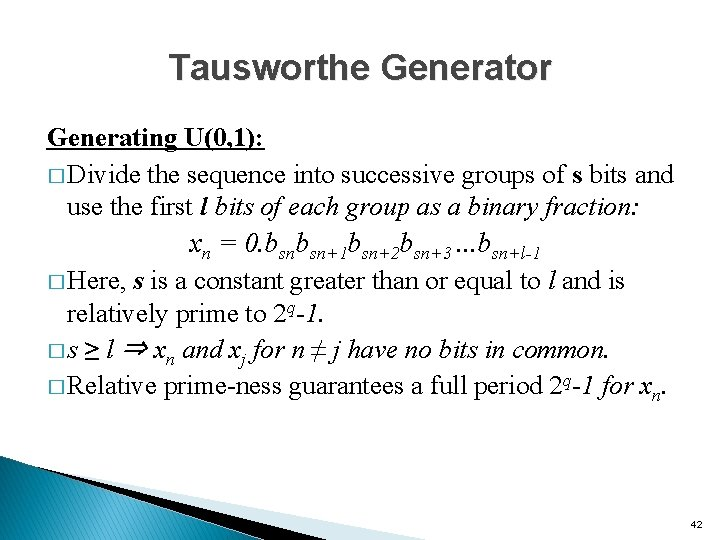Tausworthe Generator Generating U(0, 1): � Divide the sequence into successive groups of s bits and use the first l bits of each group as a binary fraction: xn = 0. bsnbsn+1 bsn+2 bsn+3. . . bsn+l-1 � Here, s is a constant greater than or equal to l and is relatively prime to 2 q-1. � s ≥ l ⇒ xn and xj for n ≠ j have no bits in common. � Relative prime-ness guarantees a full period 2 q-1 for xn. 42Tausworthe Generator Example: bn = bn-4 ⊕ bn-7 � The period 27 -1=127 � l=8, s=8: x 0 = 0. 111111102 = 0. 9921910 x 1 = 0. 000111012 = 0. 1132810 x 2 = 0. 111001012 = 0. 8945310 x 3 = 0. 100100102 = 0. 2968810 x 4 = 0. 000001002 = 0. 3632810 x 5 = 0. 010011002 = 0. 4218810 …. 43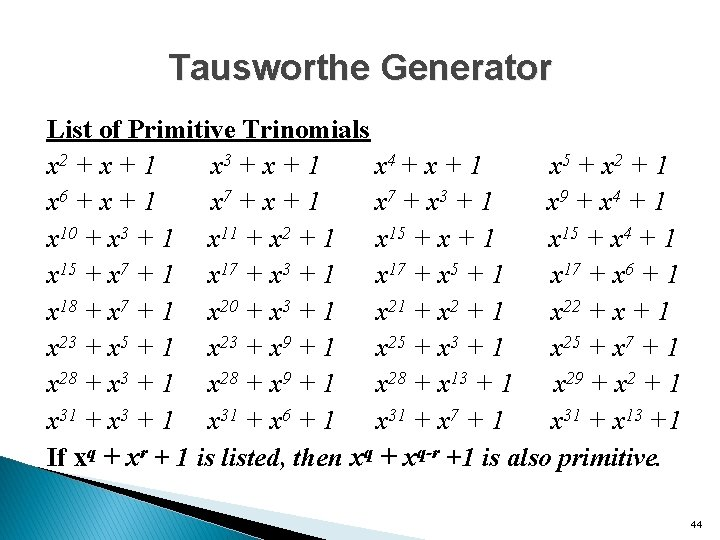Tausworthe Generator List of Primitive Trinomials x 2 + x + 1 x 3 + x + 1 x 4 + x + 1 x 5 + x 2 + 1 x 6 + x + 1 x 7 + x 3 + 1 x 9 + x 4 + 1 x 10 + x 3 + 1 x 11 + x 2 + 1 x 15 + x 4 + 1 x 15 + x 7 + 1 x 17 + x 3 + 1 x 17 + x 5 + 1 x 17 + x 6 + 1 x 18 + x 7 + 1 x 20 + x 3 + 1 x 21 + x 2 + 1 x 22 + x + 1 x 23 + x 5 + 1 x 23 + x 9 + 1 x 25 + x 3 + 1 x 25 + x 7 + 1 x 28 + x 3 + 1 x 28 + x 9 + 1 x 28 + x 13 + 1 x 29 + x 2 + 1 x 31 + x 3 + 1 x 31 + x 6 + 1 x 31 + x 7 + 1 x 31 + x 13 +1 If xq + xr + 1 is listed, then xq + xq-r +1 is also primitive. 44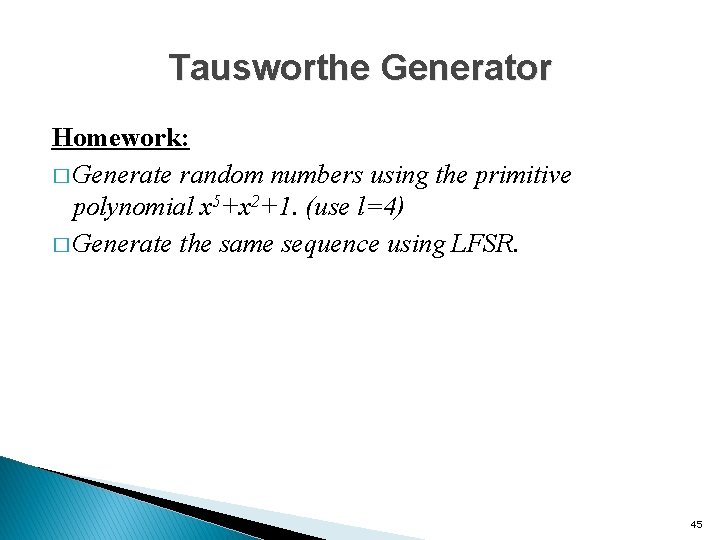Tausworthe Generator Homework: � Generate random numbers using the primitive polynomial x 5+x 2+1. (use l=4) � Generate the same sequence using LFSR. 45